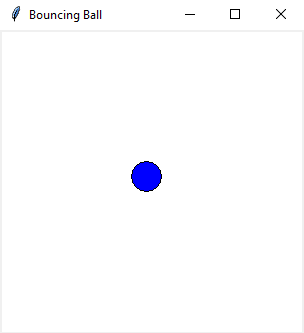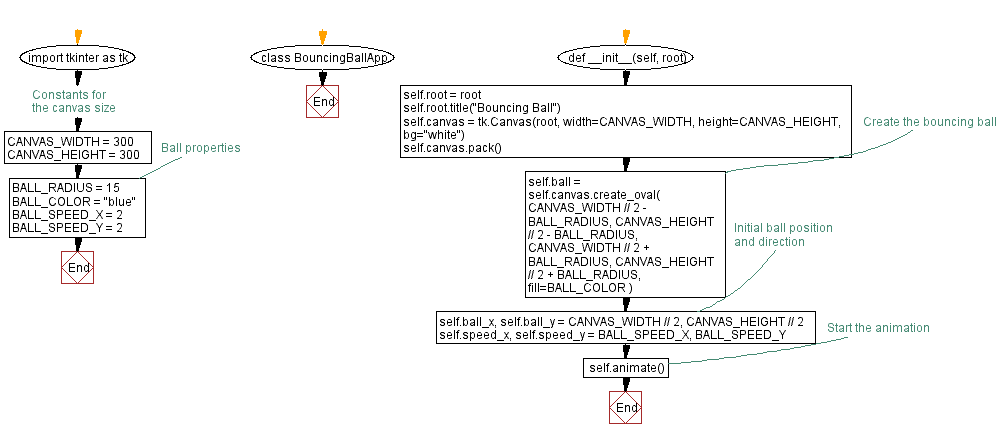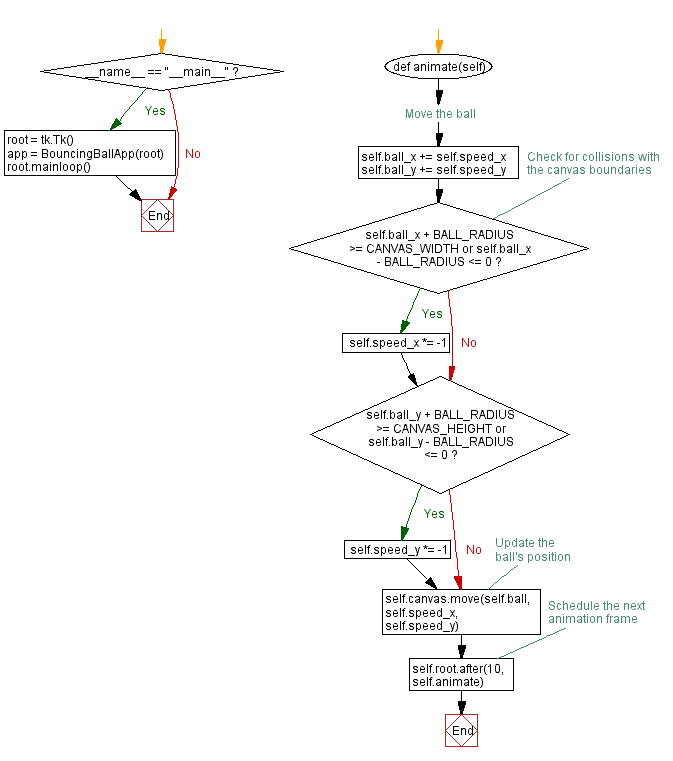﻿ Python Tkinter bouncing ball animation

# Creating a bouncing ball animation with Python and Tkinter

## Python Tkinter Canvas and Graphics: Exercise-6 with Solution

Write a Python program that uses the Canvas widget to create a simple animation, such as a bouncing ball using Tkinter.

Sample Solution:

Python Code:

``````import tkinter as tk

# Constants for the canvas size
CANVAS_WIDTH = 300
CANVAS_HEIGHT = 300

# Ball properties
BALL_COLOR = "blue"
BALL_SPEED_X = 2
BALL_SPEED_Y = 2

class BouncingBallApp:
def __init__(self, root):
self.root = root
self.root.title("Bouncing Ball")

self.canvas = tk.Canvas(root, width=CANVAS_WIDTH, height=CANVAS_HEIGHT, bg="white")
self.canvas.pack()

# Create the bouncing ball
self.ball = self.canvas.create_oval(
fill=BALL_COLOR
)

# Initial ball position and direction
self.ball_x, self.ball_y = CANVAS_WIDTH // 2, CANVAS_HEIGHT // 2
self.speed_x, self.speed_y = BALL_SPEED_X, BALL_SPEED_Y

# Start the animation
self.animate()

def animate(self):
# Move the ball
self.ball_x += self.speed_x
self.ball_y += self.speed_y

# Check for collisions with the canvas boundaries
if self.ball_x + BALL_RADIUS >= CANVAS_WIDTH or self.ball_x - BALL_RADIUS <= 0:
self.speed_x *= -1
if self.ball_y + BALL_RADIUS >= CANVAS_HEIGHT or self.ball_y - BALL_RADIUS <= 0:
self.speed_y *= -1

# Update the ball's position
self.canvas.move(self.ball, self.speed_x, self.speed_y)

# Schedule the next animation frame
self.root.after(10, self.animate)

if __name__ == "__main__":
root = tk.Tk()
app = BouncingBallApp(root)
root.mainloop()
```
```

Explanation:

In the exercise above -

• Create a Tkinter canvas and draw a red bouncing ball on it.
• The ball moves within the canvas, bouncing off the boundaries.
• The animation is achieved by repeatedly updating the ball's position and direction using the animate method and the after method to schedule the next frame.

Output:

``````

Flowchart:Python Code Editor:

What is the difficulty level of this exercise?

Test your Programming skills with w3resource's quiz.

﻿

## Python: Tips of the Day

Summing a sequence of numbers (calculating the sum of zero to ten with skips):

```>>> l = range(0,10,2)
>>> sum(l)
20
```

We are closing our Disqus commenting system for some maintenanace issues. You may write to us at reach[at]yahoo[dot]com or visit us at Facebook